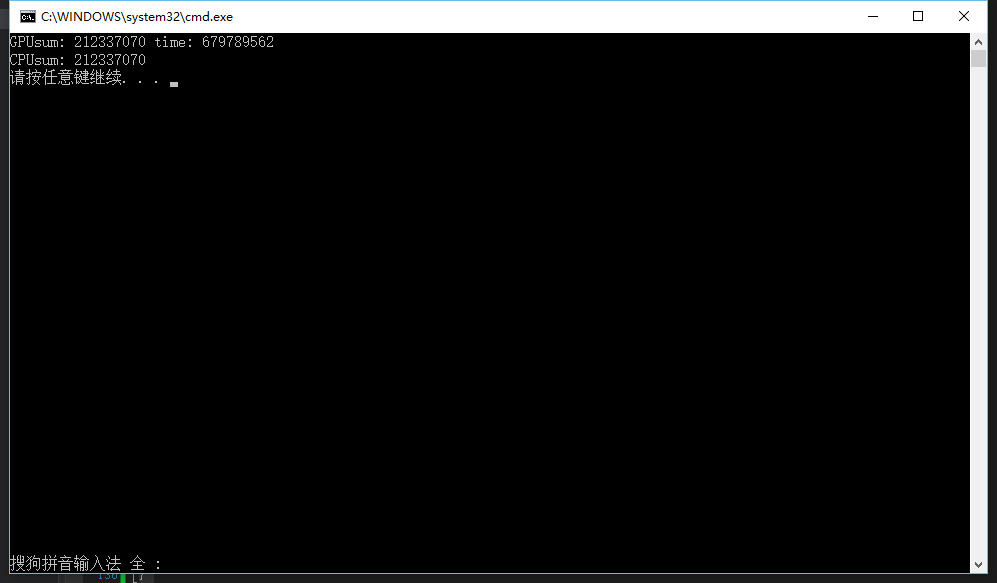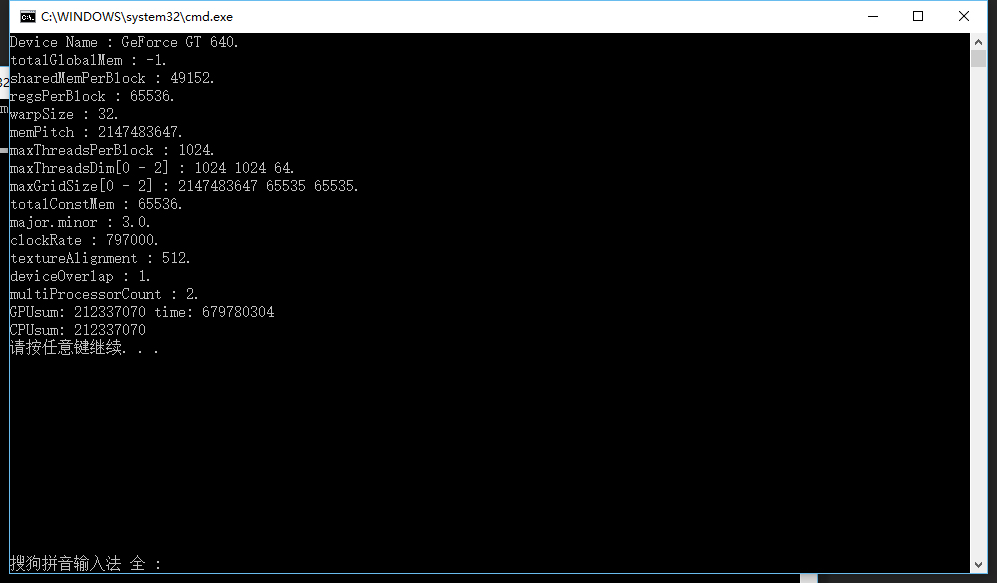# CUDA编程（三）

## 评估CUDA程序的表现

### 计算核函数运行时间

#### clock函数


#include <stdio.h>
#include <stdlib.h>

//CUDA RunTime API
#include <cuda_runtime.h>

#define DATA_SIZE 1048576

int data[DATA_SIZE];

//产生大量0-9之间的随机数
void GenerateNumbers(int *number, int size)
{
for (int i = 0; i < size; i++) {
number[i] = rand() % 10;
}
}

//CUDA 初始化
bool InitCUDA()
{
int count;

//取得支持Cuda的装置的数目
cudaGetDeviceCount(&count);

if (count == 0) {
fprintf(stderr, "There is no device.\n");
return false;
}

int i;

for (i = 0; i < count; i++) {
if (cudaGetDeviceProperties(&prop, i) == cudaSuccess) {
if (prop.major >= 1) {
break;
}
}
}

if (i == count) {
fprintf(stderr, "There is no device supporting CUDA 1.x.\n");
return false;
}

cudaSetDevice(i);

return true;
}

// __global__ 函数 (GPU上执行) 计算立方和
__global__ static void sumOfSquares(int *num, int* result)
{
int sum = 0;

int i;

for (i = 0; i < DATA_SIZE; i++) {

sum += num[i] * num[i] * num[i];

}

*result = sum;

}

int main()
{

//CUDA 初始化
if (!InitCUDA()) {
return 0;
}

//生成随机数
GenerateNumbers(data, DATA_SIZE);

/*把数据复制到显卡内存中*/

int* gpudata, *result;

//cudaMalloc 取得一块显卡内存 ( 其中result用来存储计算结果 )
cudaMalloc((void**)&gpudata, sizeof(int)* DATA_SIZE);
cudaMalloc((void**)&result, sizeof(int));

//cudaMemcpy 将产生的随机数复制到显卡内存中
//cudaMemcpyHostToDevice - 从内存复制到显卡内存
//cudaMemcpyDeviceToHost - 从显卡内存复制到内存
cudaMemcpy(gpudata, data, sizeof(int)* DATA_SIZE, cudaMemcpyHostToDevice);

// 在CUDA 中执行函数 语法：函数名称<<<block 数目, thread 数目, shared memory 大小>>>(参数...);
sumOfSquares << <1, 1, 0 >> >(gpudata, result);

/*把结果从显示芯片复制回主内存*/

int sum;

//cudaMemcpy 将结果从显存中复制回内存
cudaMemcpy(&sum, result, sizeof(int), cudaMemcpyDeviceToHost);

//Free
cudaFree(gpudata);
cudaFree(result);

printf("GPUsum: %d \n", sum);

sum = 0;

for (int i = 0; i < DATA_SIZE; i++) {
sum += data[i] * data[i] * data[i];
}

printf("CPUsum: %d \n", sum);

return 0;
}


#include <time.h>

// __global__ 函数 (GPU上执行) 计算立方和
__global__ static void sumOfSquares(int *num, int* result, clock_t* time)
{
int sum = 0;

int i;

clock_t start = clock();

for (i = 0; i < DATA_SIZE; i++) {

sum += num[i] * num[i] * num[i];

}

*result = sum;

*time = clock() - start;

}

/*把数据复制到显卡内存中*/

int* gpudata, *result;
clock_t* time;

//cudaMalloc 取得一块显卡内存 ( 其中result用来存储计算结果，time用来存储运行时间 )
cudaMalloc((void**)&gpudata, sizeof(int)* DATA_SIZE);
cudaMalloc((void**)&result, sizeof(int));
cudaMalloc((void**) &time, sizeof(clock_t));


sumOfSquares<<<1, 1, 0>>>(gpudata, result, time);

/*把结果从显示芯片复制回主内存*/

int sum;
clock_t time_used;

//cudaMemcpy 将结果从显存中复制回内存
cudaMemcpy(&sum, result, sizeof(int), cudaMemcpyDeviceToHost);
cudaMemcpy(&time_used, time, sizeof(clock_t), cudaMemcpyDeviceToHost);

//Free
cudaFree(gpudata);
cudaFree(result);
cudaFree(time);

printf("GPUsum: %d time: %d\n", sum, time_used);

#### 完整程序：


#include <stdio.h>
#include <stdlib.h>
#include <time.h>

//CUDA RunTime API
#include <cuda_runtime.h>

#define DATA_SIZE 1048576

int data[DATA_SIZE];

//产生大量0-9之间的随机数
void GenerateNumbers(int *number, int size)
{
for (int i = 0; i < size; i++) {
number[i] = rand() % 10;
}
}

//CUDA 初始化
bool InitCUDA()
{
int count;

//取得支持Cuda的装置的数目
cudaGetDeviceCount(&count);

if (count == 0) {
fprintf(stderr, "There is no device.\n");
return false;
}

int i;

for (i = 0; i < count; i++) {
if (cudaGetDeviceProperties(&prop, i) == cudaSuccess) {
if (prop.major >= 1) {
break;
}
}
}

if (i == count) {
fprintf(stderr, "There is no device supporting CUDA 1.x.\n");
return false;
}

cudaSetDevice(i);

return true;
}

// __global__ 函数 (GPU上执行) 计算立方和
__global__ static void sumOfSquares(int *num, int* result, clock_t* time)
{
int sum = 0;

int i;

clock_t start = clock();

for (i = 0; i < DATA_SIZE; i++) {

sum += num[i] * num[i] * num[i];

}

*result = sum;

*time = clock() - start;

}

int main()
{

//CUDA 初始化
if (!InitCUDA()) {
return 0;
}

//生成随机数
GenerateNumbers(data, DATA_SIZE);

/*把数据复制到显卡内存中*/
int* gpudata, *result;

clock_t* time;

//cudaMalloc 取得一块显卡内存 ( 其中result用来存储计算结果，time用来存储运行时间 )
cudaMalloc((void**)&gpudata, sizeof(int)* DATA_SIZE);
cudaMalloc((void**)&result, sizeof(int));
cudaMalloc((void**)&time, sizeof(clock_t));

//cudaMemcpy 将产生的随机数复制到显卡内存中
//cudaMemcpyHostToDevice - 从内存复制到显卡内存
//cudaMemcpyDeviceToHost - 从显卡内存复制到内存
cudaMemcpy(gpudata, data, sizeof(int)* DATA_SIZE, cudaMemcpyHostToDevice);

// 在CUDA 中执行函数 语法：函数名称<<<block 数目, thread 数目, shared memory 大小>>>(参数...);
sumOfSquares << <1, 1, 0 >> >(gpudata, result, time);

/*把结果从显示芯片复制回主内存*/

int sum;
clock_t time_used;

//cudaMemcpy 将结果从显存中复制回内存
cudaMemcpy(&sum, result, sizeof(int), cudaMemcpyDeviceToHost);
cudaMemcpy(&time_used, time, sizeof(clock_t), cudaMemcpyDeviceToHost);

//Free
cudaFree(gpudata);
cudaFree(result);
cudaFree(time);

printf("GPUsum: %d time: %d\n", sum, time_used);

sum = 0;

for (int i = 0; i < DATA_SIZE; i++) {
sum += data[i] * data[i] * data[i];
}

printf("CPUsum: %d \n", sum);

return 0;
}


#### 运行结果：（另外说一下我的环境，这里用的是Debug，后面不说明的话也是Debug下的，Release的话还会快10倍左右。然后我的显卡是NVIDIA GeForce GT 640

### 获取GPU的详细信息：

以*prop形式返回设备dev的属性。


cudaSuccess、cudaErrorInvalidDevice，注，如果之前是异步启动，该函数可能返回错误码。


struct cudaDeviceProp {

char name ;
size_t totalGlobalMem;
size_t sharedMemPerBlock;
int regsPerBlock;
int warpSize;
size_t memPitch;
int maxGridSize ;
size_t totalConstMem;
int major;
int minor;
int clockRate;
size_t textureAlignment;
int deviceOverlap;
int multiProcessorCount;

}

• name
用于标识设备的ASCII字符串;

• totalGlobalMem
设备上可用的全局存储器的总量,以字节为单位;

• sharedMemPerBlock
线程块可以使用的共享存储器的最大值,以字节为单位;多处理器上的所有线程块可以同时共享这些存储器;

• regsPerBlock
线程块可以使用的32位寄存器的最大值;多处理器上的所有线程块可以同时共享这些寄存器;

• warpSize
按线程计算的warp块大小;

• memPitch
允许通过cudaMallocPitch()为包含存储器区域的存储器复制函数分配的最大间距(pitch),以字节为单位;

每个块中的最大线程数

块各个维度的最大值:

• maxGridSize
网格各个维度的最大值;

• totalConstMem
设备上可用的不变存储器总量,以字节为单位;

• major,minor
定义设备计算能力的主要修订号和次要修订号;

• clockRate
以千赫为单位的时钟频率;

• textureAlignment
对齐要求;与textureAlignment字节对齐的纹理基址无需对纹理取样应用偏移;

• deviceOverlap
如果设备可在主机和设备之间并发复制存储器,同时又能执行内核,则此值为 1;否则此值为 0;

• multiProcessorCount
设备上多处理器的数量。

void printDeviceProp(const cudaDeviceProp &prop)
{
printf("Device Name : %s.\n", prop.name);
printf("totalGlobalMem : %d.\n", prop.totalGlobalMem);
printf("sharedMemPerBlock : %d.\n", prop.sharedMemPerBlock);
printf("regsPerBlock : %d.\n", prop.regsPerBlock);
printf("warpSize : %d.\n", prop.warpSize);
printf("memPitch : %d.\n", prop.memPitch);
printf("maxGridSize[0 - 2] : %d %d %d.\n", prop.maxGridSize, prop.maxGridSize, prop.maxGridSize);
printf("totalConstMem : %d.\n", prop.totalConstMem);
printf("major.minor : %d.%d.\n", prop.major, prop.minor);
printf("clockRate : %d.\n", prop.clockRate);
printf("textureAlignment : %d.\n", prop.textureAlignment);
printf("deviceOverlap : %d.\n", prop.deviceOverlap);
printf("multiProcessorCount : %d.\n", prop.multiProcessorCount);
}


//CUDA 初始化
bool InitCUDA()
{
int count;

//取得支持Cuda的装置的数目
cudaGetDeviceCount(&count);

if (count == 0) {
fprintf(stderr, "There is no device.\n");
return false;
}

int i;

for (i = 0; i < count; i++) {

cudaGetDeviceProperties(&prop, i);
//打印设备信息
printDeviceProp(prop);

if (cudaGetDeviceProperties(&prop, i) == cudaSuccess) {
if (prop.major >= 1) {
break;
}
}
}

if (i == count) {
fprintf(stderr, "There is no device supporting CUDA 1.x.\n");
return false;
}

cudaSetDevice(i);

return true;
}679680304 / (797000 * 1000) = 0.853S

### 计算使用的内存带宽：

1M 个 32 bits 数字的数据量是 4MB。

4MB / 0.853S = 4.68MB/s

### 什么是优秀的CUDA程序：

• 在给定的数据规模下，选用算法的计算复杂度不明显高于最优的算法；

• Active warp的数量能够让SM满载，并且active block的数量大于2，能够有效地隐藏访存延迟（使用足够大的内存带宽）；

• 当瓶颈出现在运算指令时，指令流的效率已经过了充分优化；

• 当瓶颈出现在访问IO时，程序已经选用了恰当的存储器来储存数据，并使用了适当的存储器访问方式，以获得最大带宽；

### CUDA程序编写优化步骤：

• 确定任务中的串行和并行的部分，选择合适的算法（首先将问题分解为几个步骤，确定哪些步骤可以用并行实现，并确定合适的算法）；

• 按照算法确定数据和任务的划分方式，将每个需要实现的步骤映射为一个满足CUDA两层并行模型的内核函数，让每个SM上至少有6个活动warp和至少2个活动block；

• 编写一个能正确运行的程序作为优化的起点，要确保程序能稳定运行以及其正确性，在精度不足或者发生溢出时必须使用双精度浮点或者更长的整数类型；

• 优化显存访问，避免显存带宽成为瓶颈。在显存带宽得到完全优化前，其他优化不会产生明显效果。

• 优化指令流，在误差可接受的情况下，使用CUDA算术指令集中的快速指令；避免多余的同步；在只需要少量线程进行操作的情况下，使用类似“if threaded<N”的方式，避免多个线程同时运行占用更长时间或者产生错误结果；

• 资源均衡，调整每个线程处理的数据量，shared memory和register和使用量；通过调整block大小，修改算法和指令以及动态分配shared memory，都可以提高shared的使用效率；register的多少是由内核程序中使用寄存器最多的时刻的用量决定的，因此减小register的使用相对困难；节约register方法是使用shared memory存储变量；使用括号明确地表示每个变量的生存周期；使用占用寄存器较小的等效指令代替原有指令；

• 与主机通信优化，尽量减少CPU与GPU间的传输，使用cudaMallocHost分配主机端存储器，可以获得更大带宽；一次缓存较多的数据后再一次传输，可以获得较高的带宽；需要将结果显示到屏幕的时候，直接使用与图形学API互操作的功能；使用流和异步处理隐藏与主机的通信时间；使用zero-memory技术和Write-Combined memory提高可用带宽；

### 总结：

04-06217
12-0961207-17498
08-22735
06-112340
03-31192
02-241604
04-0559